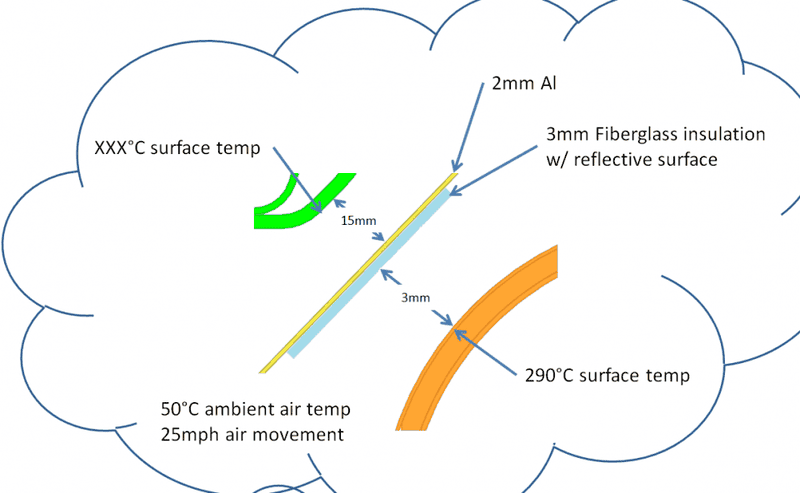# Heat transfer through AL shield by radiation & convection

• RekhaP87
In summary, the person is looking for help with a problem involving surface temperature. They are not sure if it is heat transfer (radiation), heat transfer (conduction), or heat transfer (convection) that they need to worry about, but they are familiar with the equations for each. They have taken a few heat transfer classes, and are familiar with the thermal-resistive circuit method. They are also having trouble adding convection to the problem of radiation from the heat source. They need to know the thermal resistance between the heat source and some point that they consider to be at a fixed temperature, and they also need to know more about the thermal properties of the surface they are working with.f

#### RekhaP87

Hi all,

I am new here, and want to thank you for help in advance! I am working on a project and need some help - I need to figure out surface temp on an object at some distance from the heat source:I need some help getting started.

Is it going to be: Heat transfer (radiation) + Heat transfer (conduction fiberglass) + Heat transfer conduction (Al + Heat transfer (radiation)

How do take into account convection to find surface temp at reflective surface and surface temp at green surface?

I am assuming I know the following: h(convection) air, k (fiberglass), k (Al).

Thanks,
Rp

This is a relatively complicated problem to solve, have you taken a course in Heat Transfer? What have you done so far to try and solve the problem?

This would be solved analytically using the thermal-resistive circuit method, calculating the "thermal resistance" for each part of the problem and then solving for temperature drops across each resistor. Are you familiar with this method?

This is a relatively complicated problem to solve, have you taken a course in Heat Transfer? What have you done so far to try and solve the problem?

This would be solved analytically using the thermal-resistive circuit method, calculating the "thermal resistance" for each part of the problem and then solving for temperature drops across each resistor. Are you familiar with this method?

Hi, thank you for your response! I have taken few heat transfer classes, and am familiar with the thermal-resistive circuit method. I know basic radiation, conduction, and convection equations has well.

25mph air is throwing me off in how to add the convection contribution + radiation from the heat source to the reflective material.

To calculate the contribution of of multiple heat transfer methods, you only need to calculate each method's thermal resistance and then add them as if they were parallel resistors. See attached diagram. When calculating the thermal resistance for convection, you need to calculate the convective coefficient $h$, which uses the speed of the flow as an input.

The equations you'll need to use are:
• Thermal Resistance, Conduction: $R = \frac{L}{k A}$
• Thermal Resistance, Convection: $R = \frac{1}{h A}$
• Thermal Resistance, Radiation: $R = \frac{1}{h_r A}$
Make sure and take view factors into account when you're calculating your radiation transfer coefficients.

#### Attachments

I think you will need to know more about the thermal properties of the surface who's temperature you are trying to calculate. For example the thermal resistance between it an some point that you consider to be at a fixed temperature.

There is not enough information in the initial description of the problem to allow of any meaningful calculations .

Post a sketch showing the actual configuration in 3D and tell us more about that heat source .